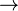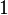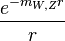# Fundamental interaction

In physics, a fundamental interaction or fundamental force is a mechanism by which particles interact with each other, and which cannot be explained in terms of another interaction. Currently, it is believed that there are four fundamental interactions: Strong interaction, weak interaction, gravitation, and electromagnetism.

## Overview

In the conceptual model of fundamental interactions, matter consists of fermions, which carry properties called charges and spin 1/2 (intrinsic angular momentum ±ℏ/2, where h/2π is reduced Planck's constant). They attract or repel each other by exchanging bosons.

The interaction of any pair of matter particles can then be modeled this way:

two fermions go in$\rightarrow$ interaction by boson exchange$\rightarrow$ two changed fermions go out.

The exchange of bosons always carries energy and momentum between the fermions, thereby changing their directions of flight and their respective speed. It may transport a charge between the fermions, changing the charges of the fermions in the process (for example, turn them from one type of fermion to another type of fermion). Since bosons carry one unit of angular momentum, the fermion's spin direction will flip from +1/2 to −1/2 (or vice versa) during such an exchange (in units of reduced Planck's constant).

Because fermions can attract and repel each other due to an interaction, such an interaction is sometimes called a "force."

Efforts of modern physics are directed at explaining every observed physical phenomenon by these interactions. Moreover, one tries to reduce the number of different interaction types (like unifying the electromagnetic interaction and the weak interaction into the electroweak interaction, see below). For an introductory explanation, four fundamental interactions (forces) may be assumed: gravitation, electromagnetism, the weak interaction, and the strong interaction. Their magnitude and behavior vary greatly, as described in the table below. Both magnitude ("relative strength") and "range," as given in the table, have some meaning only within a rather complex framework of ideas.

It should be noted that the table below lists properties of a conceptual model that is still subject to research in modern physics.

Interaction Current Theory Mediators Relative Strength Long-Distance Behavior Range(m)
Strong Quantum chromodynamics
(QCD)
gluons 1038${1}$
(see discussion below)
10-15
Electromagnetic Quantum electrodynamics
(QED)
photons 1036$\frac{1}{r^2}$ infinite
Weak Electroweak Theory W and Z bosons 1025$\frac{e^{-m_{W,Z}r}}{r}$ 10-18
Gravitation General Relativity
(GR)
gravitons (not yet discovered) 1$\frac{1}{r^2}$ infinite

The modern quantum mechanical view of the three fundamental forces (all except gravity) is that particles of matter (fermions) do not directly interact with each other, but rather carry a charge, and exchange virtual particles (gauge bosons), which are the interaction carriers or force mediators. For example, photons are the mediators of the interaction of electric charges; and gluons are the mediators of the interaction of color charges.

## The interactions

### Gravitation

Gravitation is by far the weakest interaction, but at long distances, gravity's strength relative to other forces becomes important. There are three reasons for this. First, gravity has an infinite range, like that of electromagnetism. Secondly, all masses are positive and therefore gravity's interaction cannot be screened like in electromagnetism. Finally, gravitational force cannot be absorbed or transformed, and so is permanent. Thus, large celestial bodies such as planets, stars and galaxies dominantly feel gravitational forces. In comparison, the total electric charge of these bodies is zero because half of all charges are negative. In addition, unlike the other interactions, gravity acts universally on all matter. There are no objects that lack a gravitational "charge."

Because of its long range, gravity is responsible for such large-scale phenomena as the structure of galaxies, black holes and the expansion of the universe, as well as more elementary astronomical phenomena like the orbits of planets, and everyday experience: objects fall; heavy objects act as if they were glued to the ground; people are limited in how high they can jump.

Gravitation was the first kind of interaction which was described by a mathematical theory. In ancient times, Aristotle theorized that objects of different masses fall at different rates. During the Scientific Revolution, Galileo Galilei experimentally determined that this was not the case—if friction due to air resistance is neglected, all objects accelerate toward the ground at the same rate. Isaac Newton's law of Universal Gravitation (1687) was a good approximation of the general behaviour of gravity. In 1915, Albert Einstein completed the General Theory of Relativity, a more accurate description of gravity in terms of the geometry of space-time.

An area of active research today involves merging the theories of general relativity and quantum mechanics into a more general theory of quantum gravity. It is widely believed that in a theory of quantum gravity, gravity would be mediated by a massless spin 2 particle which is known as the graviton. Gravitons are hypothetical particles not yet observed.

Although general relativity appears to present an accurate theory of gravity in the non-quantum mechanical limit, there are a number of alternate theories of gravity. Those under any serious consideration by the physics community all reduce to general relativity in some limit, and the focus of observational work is to establish limitations on what deviations from general relativity are possible.

### Electromagnetism

Main article: Electromagnetism

Electromagnetism is the force that acts between electrically charged particles. This phenomenon includes the electrostatic force, acting between charges at rest, and the combined effect of electric and magnetic forces acting between charges moving relative to each other.

Electromagnetism is also an infinite-ranged force, but it is much stronger than gravity, and therefore describes almost all phenomena of our everyday experience, ranging from the impenetrability of macroscopic bodies, to lasers and radios, to the structure of atoms and metals, to phenomena such as friction and rainbows.

Electrical and magnetic phenomena have been observed since ancient times, but it was only in the 1800s that scientists discovered that electricity and magnetism are two aspects of the same fundamental interaction. By 1864, Maxwell's equations had rigorously quantified the unified phenomenon. In 1905, Einstein's theory of special relativity resolved the issue of the constancy of the speed of light, and Einstein also explained the photoelectric effect by theorizing that light was transmitted in quanta, which we now call photons. Starting around 1927, Paul Dirac unified quantum mechanics with the relativistic theory of electromagnetism; the theory of quantum electrodynamics was completed in the 1940s by Richard Feynman, Freeman Dyson, Julian Schwinger, and Sin-Itiro Tomonaga.

### Weak interaction

The weak interaction, or weak nuclear force, is responsible for some phenomena at the scales of the atomic nucleus, such as beta decay. Electromagnetism and the weak force are theoretically understood to be two aspects of a unified electroweak interaction—this realization was the first step toward the unified theory known as the Standard Model. In electroweak theory, the carriers of the weak force are massive gauge bosons called the W and Z bosons. The weak interaction is the only known interaction in which parity is not conserved; it is left-right asymmetric. It even breaks CP symmetry. However, it does conserve CPT.

### Strong interaction

The strong interaction, or strong nuclear force, is the most complicated force because it behaves differently at different distances. At distances larger than 10 femtometers, the strong force is practically unobservable, which is why it wasn't noticed until the beginning of the 20th century.

After the nucleus was discovered, it was clear that a new force was needed to keep the positive protons in the nucleus from flying out. The force had to be much stronger than electromagnetism, so that the nucleus could be stable even though the protons were so close together, squeezed down to a volume which is 10-15 of the volume of an atom. From the short range of the force, Hideki Yukawa predicted that it was associated with a massive particle, whose mass is approximately 100 MeV. The pion was discovered in 1947 and this discovery marks the beginning of the modern era of particle physics.

Hundreds of hadrons were discovered from the 1940s to 1960s. An extremely complicated theory of the strongly interacting particles, known as hadrons, was developed. Most notably, the pions were understood to be oscillations of vacuum condensates, the rho and omega vector bosons were proposed by Sakurai to be force carrying particles for approximate symmetries of Isospin and hypercharge, and the heavier particles were grouped by Geoffrey Chew, Edward K. Burdett and Steven Frautschi into families that could be understood as vibrational and rotational excitations of strings. None of these approaches led directly to the fundamental theory, but each of these were deep insights in their own right.

Throughout the sixties, different authors considered theories similar to the modern fundamental theory of QCD as simple models for the interactions of quarks, starting with Murray Gell-Mann who along with George Zweig first proposed fractionally charged quarks in 1961. The first to suggest the gluons of QCD explicitly were the Korean physicist Moo-Young Han and Japanese Yoichiro Nambu, who introduced the quark color charge and hypothesized that it might be associated with a force-carrying field. but at that time, it was difficult to see how such a model could permanently confine quarks. Han and Nambu also assigned each quark color an integer electrical charge, so that the quarks were only fractionally charged on average, and they did not expect the quarks in their model to be permanently confined.

In 1971, Murray Gell-Mann and Harald Fritsch proposed that the Han/Nambu color gauge field was the correct theory of the short-distance interactions of fractionally charged quarks. A little later, David Gross, Frank Wilczek, and David Politzer discovered asymptotic freedom in this theory, which allowed them to make contact with experiment. They came to the conclusion that QCD was the complete theory of the strong interactions, correct at all distance scales. The discovery of asymptotic freedom led most physicists to accept QCD, since it became clear that even the long-distance properties of the strong interactions could be consistent with experiment if the quarks are permanently confined.

Assuming that quarks are confined, Mikhail Shifman, Arkady Vainshtein, and Valentine Zakharov were able to compute the properties of many low-lying hadrons directly from QCD with only a few extra parameters to describe the vacuum. First-principles computer calculations by Kenneth Wilson in 1980 established that QCD will confine quarks, to a level of confidence tantamount to certainty. From this point on, QCD was the established theory of the strong interactions.

QCD is a theory of fractionally charged quarks interacting with 8 photon-like particles called gluons. The gluons interact with each other, not just with the quarks, and at long distances the lines of force collimate into strings. In this way, the mathematical theory of QCD is not only responsible for the short-distance properties of quarks, but for the long-distance string-like behavior discovered by Chew and Frautschi.

## Current developments

The Standard Model is a theory of three fundamental forces—electromagnetism, weak interactions, and strong interactions—however, these three forces are not tied together. Howard Georgi, Sheldon Glashow, and Abdus Salam discovered that the Standard Model particles can arise from a single interaction, known as a grand unified theory. Grand unified theories predict relationships between otherwise unrelated constants of nature in the Standard Model. Gauge coupling unification is the prediction from grand unified theories for the relative strengths of the electromagnetic, weak, and strong forces, and this prediction was verified at LEP in 1991 for supersymmetric theories.

Currently, there is no complete theory of quantum gravity. There are several candidates for a framework to fit quantum gravity, including string theory, loop quantum gravity and twistor theory.

In theories beyond the Standard Model, there are frequently fifth forces and the search for these forces is an on-going line of experimental research in physics. In supersymmetric theories, there are particles that only acquire their masses through supersymmetry breaking effects and these particles, known as moduli can mediate new forces. Another possible motivation for new forces is related to the accelerating expansion of the universe. The most concrete examples of new forces from the cosmological expansion result from modifications of General Relativity.